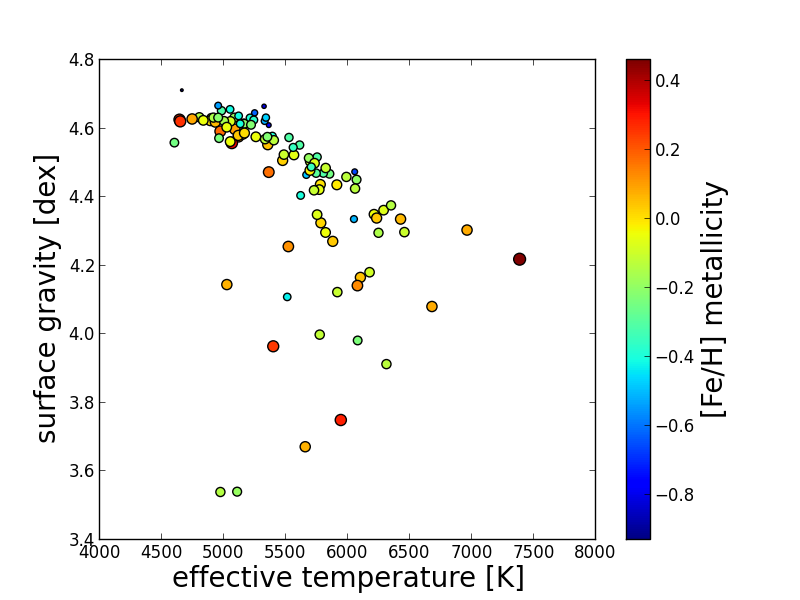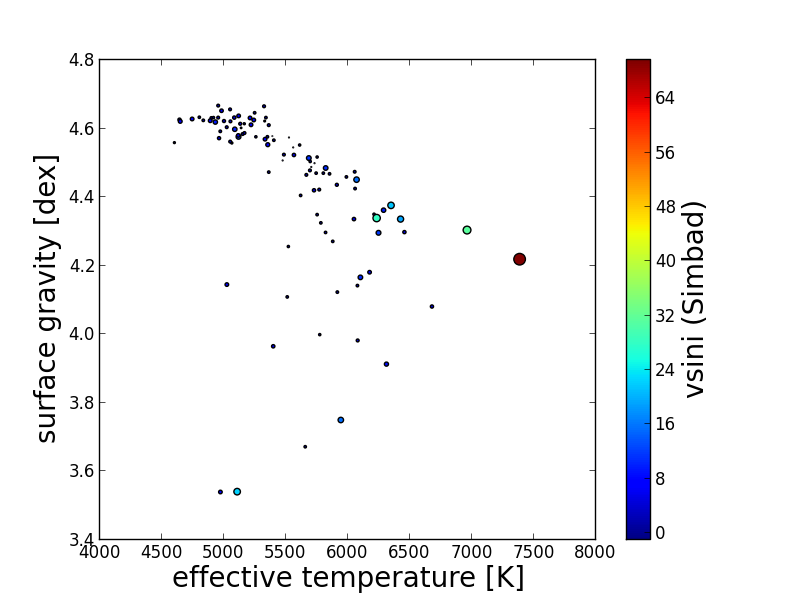VO python tutorial
Updated automatically every 5 minutes

# Using Virtual Observatory with python: querying remote astronomical databases

Frédéric Paletou, Ivan Zolotukhin (Observatoire Midi-Pyrénées, IRAP, Université Paul Sabatier - Toulouse, France)

This basic example is devoted to extending an existing catalogue with data taken elsewhere, either from CDS VizieR or Simbad database. We use the so-called “Spectroscopic Survey of  Stars in the Solar Neighborhood” (aka. S4N, Allende Prieto et al. 2004; referenced as J/A+A/420/183 in VizieR) in order to retrieve all objects with available data for the main set of stellar parameters: effective temperature (Teff), surface gravity (logg) and metallicity ([Fe/H]). Then for each object in this dataset we query Simbad database to retrieve the projected rotational velocity (vsini). This combines Vizier and Simbad queries made using python’s astroquery module. Compiling this collection of data is important for many problems in stellar astrophysics, such as the test of a stellar parameter inversion method. The tutorial covers remote database access, filtering tables with arbitrary criteria, creating and writing your own tables and basics of plotting in python.

### 1. Initialize the Python environment

You can follow this tutorial step by step in any python shell, such as python or, more conveniently, ipython. Just copy and paste the code snippets below in your python session in the terminal. Note that you have to have matplotlib, numpy, astropy and astroquery python modules installed in your system. Do not hesitate to print any variable below (print varname) to explore data structures we use in the tutorial.

 import os#--- import pylab stuffimport pylab as pltimport numpy as np#--- import astropy stufffrom astropy.table import Table, Column#--- import astroquery stufffrom astroquery.vizier import Vizierfrom astroquery.simbad import Simbad

### 2. Proceed with the query of CDS Vizier service

The S4N catalogue contains 4 different tables, which command find_catalogs and get_catalogs allow to identify. We are interested in “table2” which is s4n hereafter.

One “trick” is to know name conventions (e.g., __Fe_H_) in order to retrieve specific stellar parameters. It may also be useful to get information on units into which they have been expressed.

 # this is the trick: tell to return column name 'all'v = Vizier(columns=['all'])catalist = Vizier.find_catalogs('J/A+A/420/183')#--- trick: in order to get all rows in tablesVizier.ROW_LIMIT = -1s4n = Vizier.get_catalogs(catalist.keys())

At this stage, one has retrieved the content of the S4N Vizier catalogue for these stellar fundamental parameters. Notice that some objects do not have the full set of these 3 stellar fundamental parameters available.

Afterwards, we shall keep only these objects for which all of these 3 parameters are set in the S4N catalogue.

### 3. Filter data

 #--- retain only targets for which all 3 parameters have been setfilter = (s4n['Teff'].mask == False) & (s4n['logg'].mask == False) & \  (s4n['__Fe_H_'].mask == False)#--- compute number of targetsntargets = len(s4n[filter])#--- we shall need to restore full HIP # for Simbad queries... objhip = []#--- apply filter to tablesobj = s4n['HIP'][filter]teff = s4n['Teff'][filter]logg = s4n['logg'][filter]mtal = s4n['__Fe_H_'][filter]

A note on units: the S4N survey expresses metallicities in “H=12” and we convert them in more usual “dex” so that the solar [Fe/H]=0.

 #--- now convert to usual (dex) metallicitymtal = mtal - 7.55

### 4. Make CDS Simbad queries

For each row in our filtered table derived from the S4N catalogue we make a Simbad query by object name (e.g. ‘HIP 171’) and save vsini value in a new array.

 #--- restoring full HIP names for next (simbad) queriesfor i in np.arange(ntargets):    objhip.append('HIP' + str(obj[i]))#--- now retrieving vsini value (most recent though) from SimbadSimbad.add_votable_fields('rot')vsini=np.zeros((ntargets), 'Float64')    for i in np.arange(1, ntargets):    t = Simbad.query_object(objhip[i])    if (len(t) <> 0):        vsini[i] = t['ROT_Vsini']    else:        vsini[i] = -1.

We start the last loop above from index 1 to make sure we avoid querying Simbad with HIP0==Sun in the S4N catalogue.

### 5. Saving result catalogue/table

This can be made using the astropy.table module.

 #--- make my own table...MyS4N = Table( [objhip, teff, logg, mtal, vsini], names=('ObjHIP','Teff','logg','[Fe/H]','vsini'))#--- save table as Fits fileMyS4N.write('MyS4N.fits')

This table may be exported in various formats (e.g. VOTable or ASCII) for the record or for data exchange between collaborators (see the astropy documentation). Here we save data as a FITS file.

### 6. A graphical summary of the output

 #--- graphical summary/outputplt.figure(1)plt.scatter(teff, logg, s=50*(mtal+1), c=mtal)plt.title('S4N (Allende Prieto et al. 2004)', fontsize=20)plt.xlabel('effective temperature [K]', fontsize=20)plt.ylabel('surface gravity [dex]', fontsize=20)cb = plt.colorbar()cb.set_label('[Fe/H] metallicity', size=20)plt.figure(2)plt.scatter(teff, logg, s=vsini, c=vsini)plt.title('S4N (Allende Prieto et al. 2004)', fontsize=20)plt.xlabel('effective temperature [K]', fontsize=20)plt.ylabel('surface gravity [dex]', fontsize=20)cb = plt.colorbar()cb.set_label('vsini (Simbad)', size=20)plt.show()Fig. 1: A summary of collected data from the S4N survey. Only data for which {Teff., logg,  [Fe/H]} are simultaneously available have been considered. Metallicities have been converted to more usual “dex” values such as the solar [Fe/H]=0.Fig. 2: Same as Fig. 1 but surface/color of the dots now vary with the projected rotational velocity vsini value retrieved from Simbad.

You can download the full Python script from this tutorial here: http://goo.gl/LmdPOI